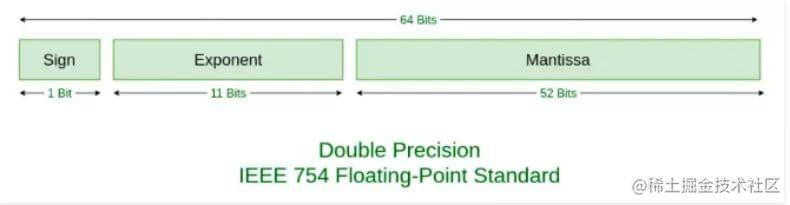# JavaScript 深入之浮点数精度## 前言

0.1 + 0.2 是否等于 0.3 作为一道经典的面试题，已经广外熟知，说起原因，大家能回答出这是浮点数精度问题导致，也能辩证的看待这并非是 ECMAScript 这门语言的问题，今天就是具体看一下背后的原因。

## 数字类型

ECMAScript 中的 Number 类型使用 IEEE754 标准来表示整数和浮点数值。所谓 IEEE754 标准，全称 IEEE 二进制浮点数算术标准，这个标准定义了表示浮点数的格式等内容。

## 浮点数转二进制

1020 = 1 * 10^3 + 0 * 10^2 + 2 * 10^1 + 0 * 10^0

1020 = 1 * 2^9 + 1 * 2^8 + 1 * 2^7 + 1 * 2^6 + 1 * 2^5 + 1 * 2^4 + 1 * 2^3 + 1 * 2^2 + 0 * 2^1 + 0 * 2^0

0.75 = a * 2^-1 + b * 2^-2 + c * 2^-3 + d * 2^-4 + ...

0.75 = a * 2^-1 + b * 2^-2 + c * 2^-3 + d * 2^-4...

1 + 0.5 = a * 2^0 + b * 2^-1 + c * 2^-2 + d * 2^-3... (所以 a = 1)

0.5 = b * 2^-1 + c * 2^-2 + d * 2^-3...

1 + 0 = b * 2^0 + c * 2^-2 + d * 2^-3... (所以 b = 1)

``````0.1 = a * 2^-1 + b * 2^-2 + c * 2^-3 + d * 2^-4 + ...

0 + 0.2 = a * 2^0 + b * 2^-1 + c * 2^-2 + ...   (a = 0)
0 + 0.4 = b * 2^0 + c * 2^-1 + d * 2^-2 + ...   (b = 0)
0 + 0.8 = c * 2^0 + d * 2^-1 + e * 2^-2 + ...   (c = 0)
1 + 0.6 = d * 2^0 + e * 2^-1 + f * 2^-2 + ...   (d = 1)
1 + 0.2 = e * 2^0 + f * 2^-1 + g * 2^-2 + ...   (e = 1)
0 + 0.4 = f * 2^0 + g * 2^-1 + h * 2^-2 + ...   (f = 0)
0 + 0.8 = g * 2^0 + h * 2^-1 + i * 2^-2 + ...   (g = 0)
1 + 0.6 = h * 2^0 + i * 2^-1 + j * 2^-2 + ...   (h = 1)
....

## 浮点数的存储

Value = sign * exponent * fraction

-1 * 10^3 * 1.02

sign 就是 -1，exponent 就是 10^3，fraction 就是 1.02

1 * 2^-4 * 1.1001100110011……

V = (-1)^S * (1 + Fraction) * 2^E

(如果所有的浮点数都可以这样表示，那么我们存储的时候就把这其中会变化的一些值存储起来就好了)

`(-1)^S` 表示符号位，当 S = 0，V 为正数；当 S = 1，V 为负数。0 01111111011 1001100110011001100110011001100110011001100110011010

0 01111111100 1001100110011001100110011001100110011001100110011010

## 浮点数的运算

``````  0.1100110011001100110011001100110011001100110011001101
+ 1.1001100110011001100110011001100110011001100110011010
————————————————————————————————————————————————————————
10.0110011001100110011001100110011001100110011001100111

`1.0011001100110011001100110011001100110011001100110100 * 2^-2`

0 01111111101 0011001100110011001100110011001100110011001100110100

## 其他

``````// 十进制转二进制
parseFloat(0.1).toString(2);
=> "0.0001100110011001100110011001100110011001100110011001101"

// 二进制转十进制
parseInt(1100100,2)
=> 100

// 以指定的精度返回该数值对象的字符串表示
(0.1 + 0.2).toPrecision(21)
=> "0.300000000000000044409"
(0.3).toPrecision(21)
=> "0.299999999999999988898"

## 深入系列

JavaScript深入系列目录地址：github.com/mqyqingfeng…

JavaScript深入系列预计写十五篇左右，旨在帮大家捋顺JavaScript底层知识，重点讲解如原型、作用域、执行上下文、变量对象、this、闭包、按值传递、call、apply、bind、new、继承等难点概念。

• 谢小飞
5年前
•• CamSong
5年前
•• Max_Cheng
1年前•• DonV
1年前